Function Repository Resource:

# DLMFHue

Get a color based on the Digital Library of Mathematical Functions (DLMF) color wheel

Contributed by: Jan Mangaldan
 ResourceFunction["DLMFHue"][h] is a color based on the color wheel, as modified by the Digital Library of Mathematical Functions (DLMF). ResourceFunction["DLMFHue"][h,s,b] specifies the saturation and brightness. ResourceFunction["DLMFHue"][h,s,b,a] specifies opacity a.

## Details and Options

The parameters h, s, b, and a must all be between 0 and 1. Values of s, b, and a outside this range are clipped. Values of h outside this range are treated cyclically.
As h varies from 0 to 1, the color corresponding to ResourceFunction["DLMFHue"][h] runs through red, yellow, green, cyan, blue, magenta, and back to red again.
ResourceFunction["DLMFHue"][h] is equivalent to ResourceFunction["DLMFHue"][h,1,1].
The alternative forms ResourceFunction["DLMFHue"][{h,s,b}] and ResourceFunction["DLMFHue"][{h,s,b,a}] can also be used.
ResourceFunction["DLMFHue"][h,s,b,a] is equivalent to Directive[ResourceFunction["DLMFHue"][h,s,b],Opacity[a]].
If no opacity has been specified, ResourceFunction["DLMFHue"][h,s,b] is equivalent to ResourceFunction["DLMFHue"][h,s,b,1].

## Examples

### Basic Examples (2)

Specify the color of a graphic primitive:

 In:=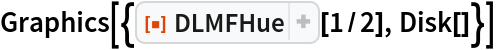Out=Specify the color with opacity:

 In:=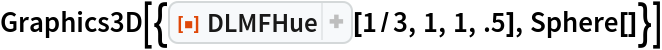Out=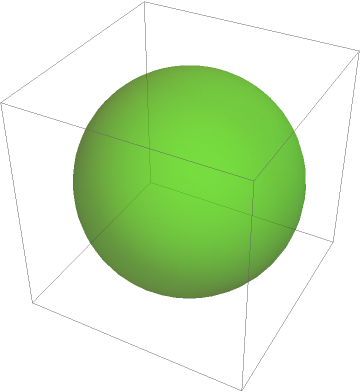### Scope (5)

Visualize the DLMF color wheel:

 In:=Out=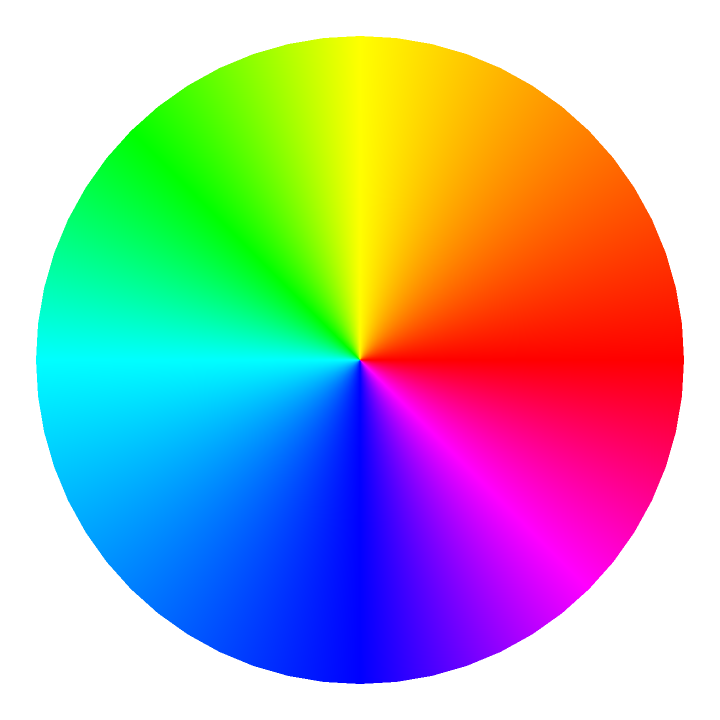DLMFHue[h] is equivalent to DLMFHue[h,1,1]:

 In:=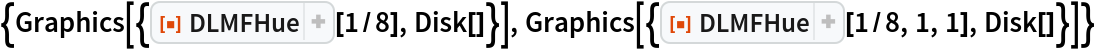Out=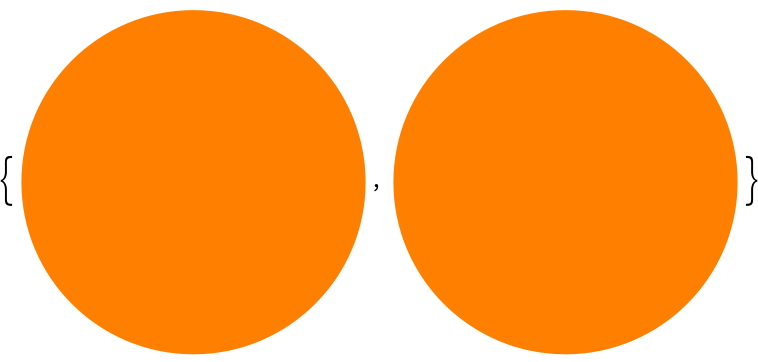DLMFHue[{h,s,b}] is equivalent to DLMFHue[h,s,b]:

 In:=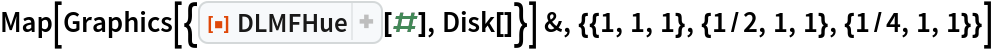Out=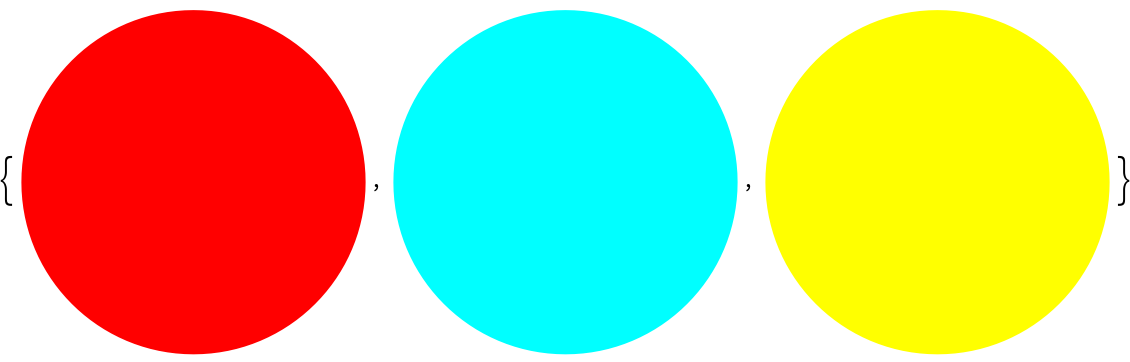Use Opacity with DLMFHue:

 In:=Out=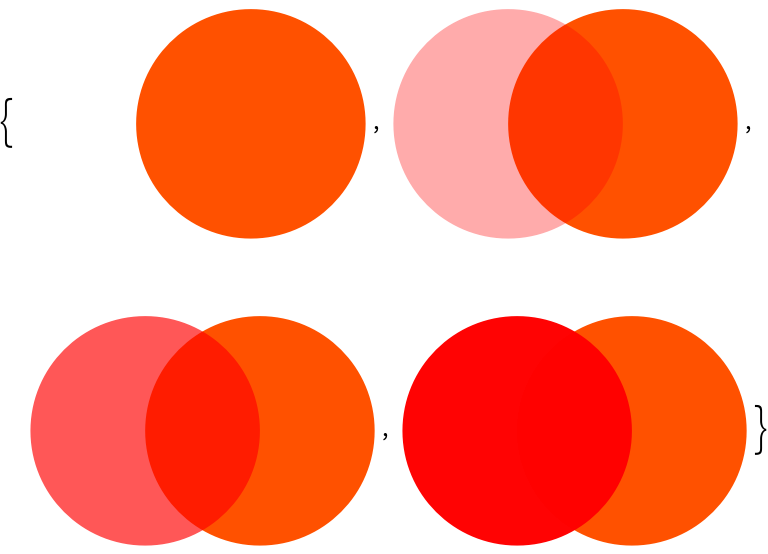Use the opacity argument in DLMFHue directly:

 In:=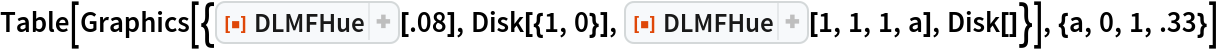Out=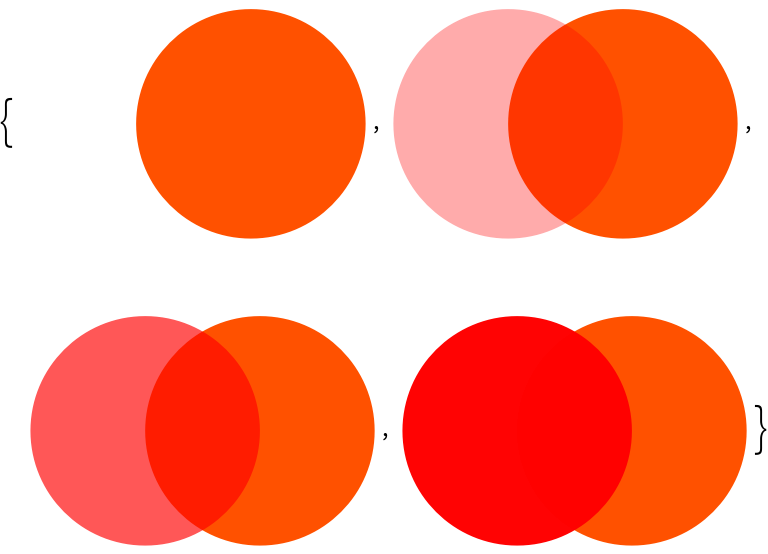### Applications (2)

Use with ComplexPlot:

 In:=Out=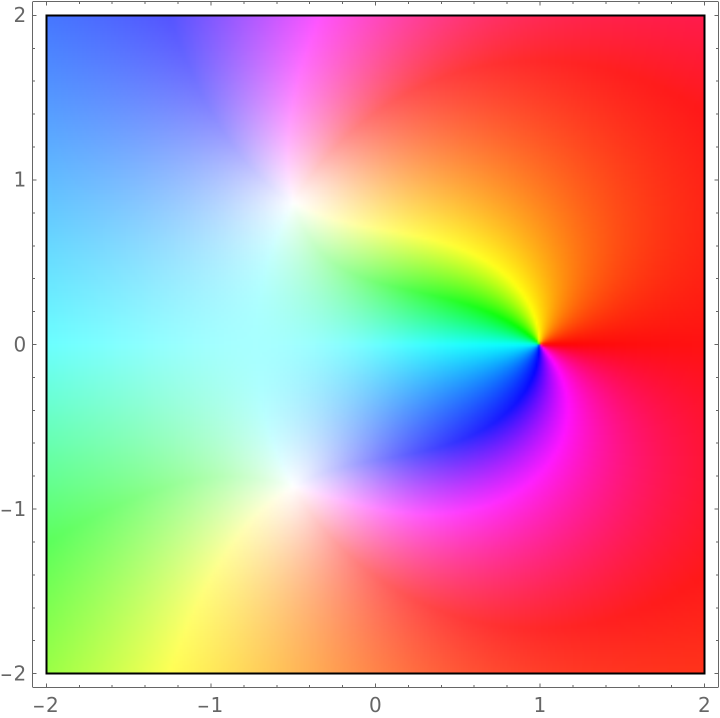Use with ComplexPlot3D:

 In:=Out=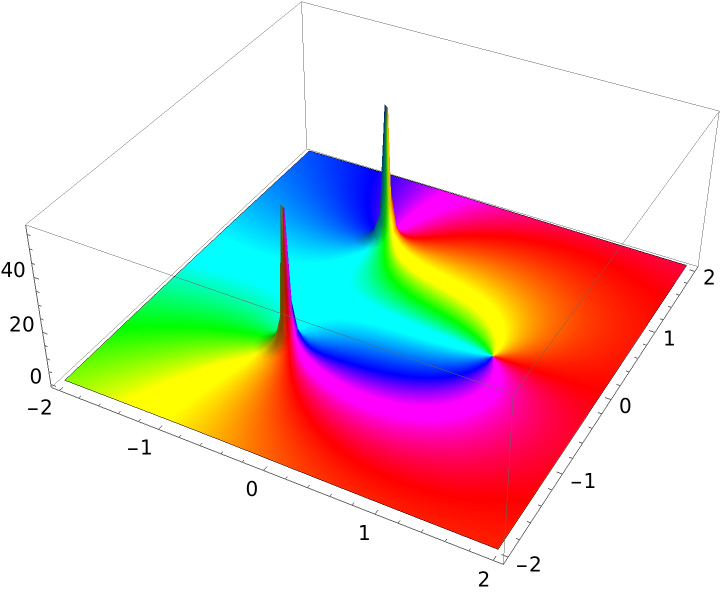### Properties and Relations (2)

DLMFHue is a transformed version of Hue:

 In:=In:=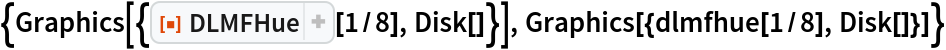Out=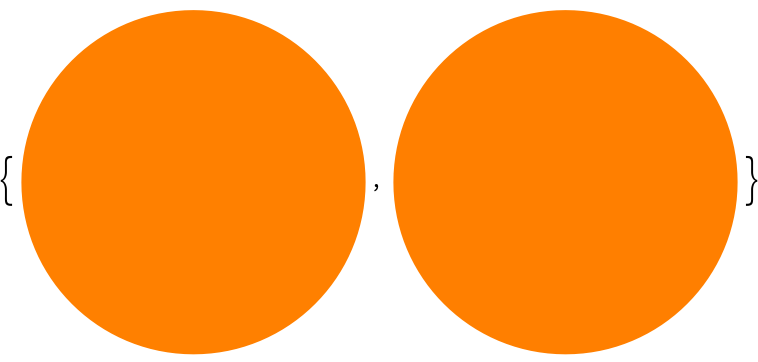In plot functions, use ColorFunctionScaling to control global scaling of variables:

 In:=Out=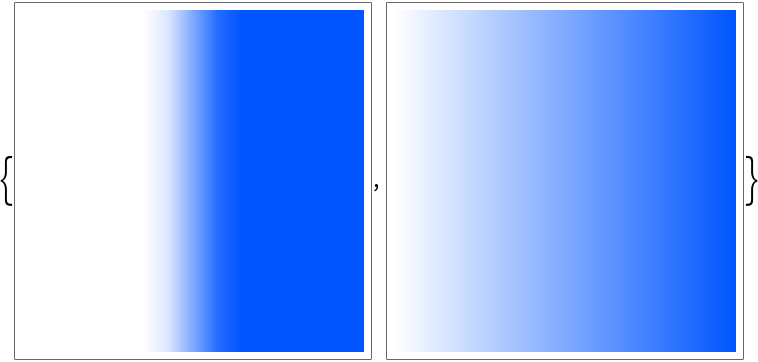### Possible Issues (1)

Saturation and brightness values outside of the {0, 1} range will be clipped:

 In:=Out=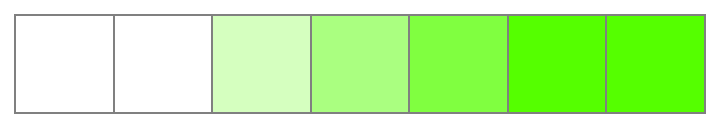In:=Out=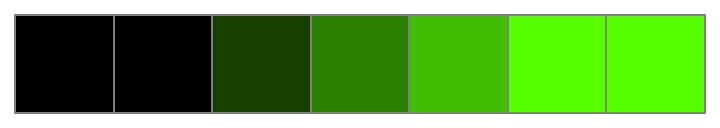## Version History

• 1.0.0 – 22 December 2020